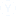# what is the syllabus of PGT Mathematics

mathematics m.sc

• Anita
• 08 oct.
• 23121 Visto
• 2 Respuestas

Syllabus for PGT Mathematics Exam:
1. Sets
2. Relations & Functions
3. Principle of Mathematical Induction
4. Permutations & Combinations
5. Complex Numbers
6. Linear Inequalities
7. Sequence and Series
8. Elementary Number Theory
10. Matrices and Determinants
11. Two dimensional Geometry
12. Trigonometric Functions
13. Inverse Trigonometric Functions
14. Differential Calculus
15. Applications of Derivatives
16. Integral Calculus
17. Differential Equations
18. Vectors
19. Three dimensional Geometry
20. Statistics
21. Probability
22. Linear AlgebraOtras discusiones relacionadasPractica simulacro de prueba para
Army Welfare Education Society (AWES) PGT TGT PRT